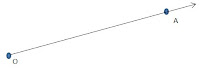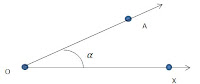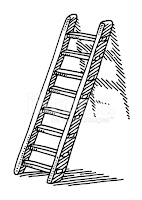## Revision Notes for Ch 9 Some Application of Trigonometry Class 10th Mathematics

Introduction

→ This chapter is an application of trigonometry. In trigonometry, we use to measure sides of triangle, when particular angle was given. Most the buildings, walls, towers we see around are perpendicular to ground. The chapter deals with measurement of heights and distance from certain points with the help of trigonometry if particular angles are known.

Line of Sight: When an observer looks from a point O at an object A, then OA is called line of sight.Angle of Elevation: Suppose that from a point O, you look up at point A, then the angle, the which the line of sight makes with the horizontal line through O is called the angle of elevation of A, as we seen from O.Here OX is horizontal line. is here angle of elevation.

Angle of depression: Suppose that from the point O, you look down at an object B, placed below the level of your eye. Then the angle which the line of sight makes with the horizontal line through O is called angle of depression of B for O.Here, OX is horizontal line. β is here angle of deviation.

An Example of Angle of Elevation and Depression

A man standing on a building will look at a building higher than his. If he sees at the top, he will look at the top he will form angle of elevation. If he look at the bottom he will form angle of depression with bottom of the building.

Solved Example based on The Concept

Q. The angle of elevation of a cloud 60m above a lake is 30° and the angle of reflection of the cloud in the lake is 60°. Find height of the cloud.

The situation is depicted by the following diagram.Let AB be the surface of the lake and let P be the point vertically above A such that AP=60m
Let C be the position of cloud and let D be its reflection in the lake.
Let the height of Cloud be H meters.
Then BC=BD=H (Let)
Draw PQ Perpendicular to CD
⇒∠QPC=30°,∠QPD=60°
BQ=AP=60m
CQ=(H-60)m,DQ=(H+60)m

From right triangle ∆ CQP, we have
Let AB be the surface of the lake and let P be the point vertically above A such that AP=60m
Let C be the position of cloud and let D be its reflection in the lake.
Let the height of Cloud be H meters.
Then BC=BD=H (Let)
Draw PQ Perpendicular to CD
⇒∠QPC=30°,∠QPD=60°
BQ=AP=60m
CQ=(H-60)m,DQ=(H+60)m
From right triangle ∆ CQP, we have
PQ/CQ=cot⁡30°
PQ/(H-60) = √3
PQ⇒(H-60) √3 m (i)
From the right triangle ∆ DQP
PQ/DQ=cot⁡60°
⇒PQ=(H+60)/√3 (ii)
From (i) and (ii), we get
(H-60) √3=(H+60)/√3
⇒3H-180=H+60
⇒H=240
So, height of the cloud is H=240m.